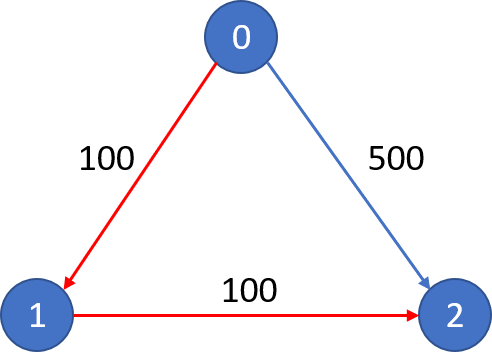787. Cheapest Flights Within K Stops

There are `n` cities connected by `m` flights. Each flight starts from city `u` and arrives at `v` with a price `w`.

Now given all the cities and flights, together with starting city `src` and the destination `dst`, your task is to find the cheapest price from `src` to `dst` with up to `k` stops. If there is no such route, output `-1`.

```Example 1:
Input:
n = 3, edges = [[0,1,100],[1,2,100],[0,2,500]]
src = 0, dst = 2, k = 1
Output: 200
Explanation:
The graph looks like this:The cheapest price from city `0` to city `2` with at most 1 stop costs 200, as marked red in the picture.```
```Example 2:
Input:
n = 3, edges = [[0,1,100],[1,2,100],[0,2,500]]
src = 0, dst = 2, k = 0
Output: 500
Explanation:
The graph looks like this:The cheapest price from city `0` to city `2` with at most 0 stop costs 500, as marked blue in the picture.
```

Constraints:

• The number of nodes `n` will be in range `[1, 100]`, with nodes labeled from `0` to `n`` - 1`.
• The size of `flights` will be in range `[0, n * (n - 1) / 2]`.
• The format of each flight will be `(src, ``dst``, price)`.
• The price of each flight will be in the range `[1, 10000]`.
• `k` is in the range of `[0, n - 1]`.
• There will not be any duplicated flights or self cycles.

787. Cheapest Flights Within K Stops
``````struct Solution;

use std::cmp::Ordering;
use std::collections::BinaryHeap;
use std::i32;

#[derive(Eq, Debug)]
struct State {
position: usize,
stop: usize,
cost: i32,
}

impl Ord for State {
fn cmp(&self, other: &Self) -> Ordering {
other.cost.cmp(&self.cost)
}
}

impl PartialOrd for State {
fn partial_cmp(&self, other: &Self) -> Option<Ordering> {
Some(self.cmp(other))
}
}

impl PartialEq for State {
fn eq(&self, other: &Self) -> bool {
self.cost == other.cost
}
}

#[derive(Copy, Clone)]
struct Edge {
u: usize,
v: usize,
cost: i32,
}

impl Solution {
fn find_cheapest_price(n: i32, flights: Vec<Vec<i32>>, src: i32, dst: i32, k: i32) -> i32 {
let n = n as usize;
let src = src as usize;
let dst = dst as usize;
let k = k as usize;
let mut prices = vec![i32::MAX; n];
prices[src] = 0;
let mut edges: Vec<Vec<Edge>> = vec![vec![]; n];
for f in flights {
let u = f as usize;
let v = f as usize;
let cost = f;
edges[u].push(Edge { u, v, cost });
}
let mut pq: BinaryHeap<State> = BinaryHeap::new();
pq.push(State {
position: src,
stop: 0,
cost: 0,
});
while let Some(s) = pq.pop() {
if s.position == dst {
return s.cost;
}
prices[s.position] = i32::min(s.cost, prices[s.position]);
if s.stop <= k {
for e in &edges[s.position] {
pq.push(State {
position: e.v,
cost: s.cost + e.cost,
stop: s.stop + 1,
});
}
}
}
if prices[dst] == i32::MAX {
-1
} else {
prices[dst]
}
}
}

#[test]
fn test() {
let n = 3;
let flights = vec![vec![0, 1, 100], vec![1, 2, 100], vec![0, 2, 500]];
let src = 0;
let dst = 2;
let k = 1;
assert_eq!(Solution::find_cheapest_price(n, flights, src, dst, k), 200);
}
``````